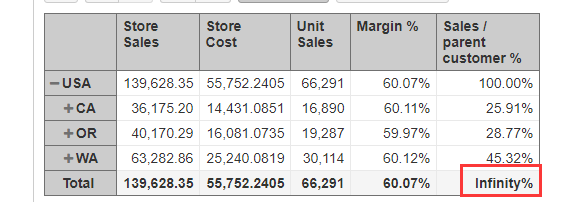# How to get rid of this wrong value

HI！
In this demo ：https://eazybi.com/accounts/1/cubes/Sales/
How to get rid of this wrong value：“Infinity%”Hi!

The “Sales / parent customer %” is dividing current Sales value with one level higher. In case of Total value there is no ‘one level higher’ value to divide with and in that case division with zero was happening giving the Infinity % result.

I have fixed the formula to divide with top level in case of Total for this measure.

``````...
WHEN [Customers].CurrentMember.Name = '\$total_aggregate'
THEN
[Measures].[Store Sales] /
([Measures].[Store Sales], [Customers].DefaultMember)
...
``````

But in this case, it is more fitting to use the hierarchy to see the totals instead of the Total sum as then the division with the parent would be more accurate.

Let me know if you have any further questions!
Lauma / support@eazybi.com

1 Like